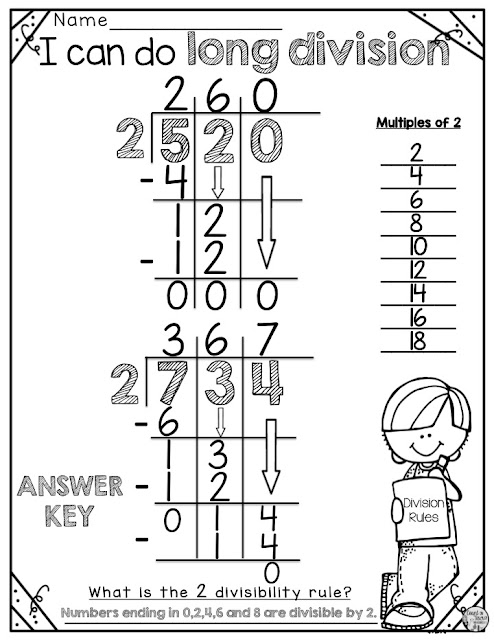## April 7, 2019

### Long Division Tips and Tricks for Beginners with a FREEBIE

Long Division....let's start with the math basics.  Ironically, I struggled in math as an elementary school student.  It wasn't until I was a teacher that I actually understood some simple math mantras.

### Count on Tricia's Math Mantras

Math Made Easy - Tip One

Math Made Easy - Tip Two
Multiplication is putting equal groups together.

Math Made Easy - Tip Three
Division is splitting apart equal groups.

Math Made Easy - Tip Four
Break numbers apart into smaller numbers and think friendly numbers. For example think MULTIPLES of 10 or 100.

Math Made Easy - Tip Five
Make a Ballpark Estimate before solving a math problem.

Math Made Easy - Tip Six
There are MANY ways to solve a math problem.  It is my job as a teacher to show students several different ways to solve a problem, but in the end, the students use which way works best for them because everyone thinks differently.

Long Division PARTIAL QUOTIENT STRATEGY
Believe it or not, many of my 4th grade students preferred using this division strategy.
This strategy is all about breaking numbers into smaller numbers and thinking friendly numbers (tip 4)
Here is an example using the number 520.  This is just one way to break 520 into smaller friendlier numbers.To grab this Long Division Partial Quotients freebie, click hereClick here to grab this Long Division Practice freebie.  (same as above)